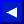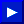### INT

TYPE: Integer Function
FORMAT: INT (<numeric>)

Action: Returns the integer value of the expression. If the expression is positive, the fractional part is left off. If the expression is negative, any fraction causes the next lower integer to be returned.

EXAMPLES of INT Function:
```   120 PRINT INT(99.4343), INT(-12.34)

99       -13
```

### LEFT\$

TYPE: String Function
FORMAT: LEFT\$ (<string>, <integer>)

Action: Returns a string comprised of the leftmost <integer> characters of the <string>. The integer argument value must be in the range 0 to 255. If the integer is greater than the length of the string, the entire string will be returned. If an <integer> value of zero is used, then a null string (of zero length) is returned.

EXAMPLES of LEFT\$ Function:
```   10 A\$ = "COMMODORE COMPUTERS"
20 B\$ = LEFT\$(A\$,9): PRINT B\$
RUN

COMMODORE
```This page has been created by Sami Rautiainen. Read the small print. Last updated December 22, 2002.